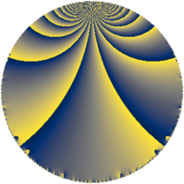# Properties

 Label 49.4.gLevel $49$ Weight $4$ Character orbit 49.g Rep. character $\chi_{49}(2,\cdot)$ Character field $\Q(\zeta_{21})$ Dimension $156$ Newform subspaces $1$ Sturm bound $18$ Trace bound $0$

# Related objects

## Defining parameters

 Level: $$N$$ $$=$$ $$49 = 7^{2}$$ Weight: $$k$$ $$=$$ $$4$$ Character orbit: $$[\chi]$$ $$=$$ 49.g (of order $$21$$ and degree $$12$$) Character conductor: $$\operatorname{cond}(\chi)$$ $$=$$ $$49$$ Character field: $$\Q(\zeta_{21})$$ Newform subspaces: $$1$$ Sturm bound: $$18$$ Trace bound: $$0$$

## Dimensions

The following table gives the dimensions of various subspaces of $$M_{4}(49, [\chi])$$.

Total New Old
Modular forms 180 180 0
Cusp forms 156 156 0
Eisenstein series 24 24 0

## Trace form

 $$156 q - 13 q^{2} - 7 q^{3} + 35 q^{4} - 7 q^{5} - 70 q^{6} - 42 q^{7} + 16 q^{8} + 198 q^{9} + O(q^{10})$$ $$156 q - 13 q^{2} - 7 q^{3} + 35 q^{4} - 7 q^{5} - 70 q^{6} - 42 q^{7} + 16 q^{8} + 198 q^{9} + 85 q^{11} - 427 q^{12} + 14 q^{13} - 203 q^{14} + 230 q^{15} + 127 q^{16} + 189 q^{17} - 16 q^{18} - 490 q^{19} - 406 q^{20} + 231 q^{21} + 590 q^{22} - 377 q^{23} - 812 q^{24} + 142 q^{25} + 490 q^{26} + 14 q^{27} - 280 q^{28} + 172 q^{29} - 39 q^{30} - 1162 q^{31} - 1099 q^{32} - 49 q^{33} + 882 q^{34} + 847 q^{35} + 812 q^{36} - 377 q^{37} - 1596 q^{38} - 770 q^{39} - 1694 q^{40} - 938 q^{41} - 3290 q^{42} - 10 q^{43} + 4354 q^{44} + 1176 q^{45} + 3408 q^{46} + 1379 q^{47} + 9604 q^{48} + 2576 q^{49} - 6184 q^{50} + 2271 q^{51} + 6874 q^{52} + 1905 q^{53} + 1617 q^{54} - 1834 q^{55} + 1736 q^{56} + 2673 q^{57} - 4716 q^{58} + 63 q^{59} - 10850 q^{60} - 2625 q^{61} - 4298 q^{62} - 1806 q^{63} - 2756 q^{64} - 196 q^{65} + 2100 q^{66} - 202 q^{67} - 5635 q^{68} - 3248 q^{69} + 6650 q^{70} + 2608 q^{71} - 6424 q^{72} - 2471 q^{73} + 2582 q^{74} + 3612 q^{75} - 4529 q^{76} - 1281 q^{77} + 5040 q^{78} - 608 q^{79} - 12348 q^{80} - 17375 q^{81} - 3234 q^{82} - 9842 q^{83} - 11564 q^{84} - 512 q^{85} - 2301 q^{86} + 2254 q^{87} + 4033 q^{88} + 4977 q^{89} + 18312 q^{90} - 8932 q^{91} + 7714 q^{92} + 14164 q^{93} + 2506 q^{94} + 11504 q^{95} + 12642 q^{96} + 11116 q^{97} + 29771 q^{98} - 10804 q^{99} + O(q^{100})$$

## Decomposition of $$S_{4}^{\mathrm{new}}(49, [\chi])$$ into newform subspaces

Label Dim $A$ Field CM Traces $q$-expansion
$a_{2}$ $a_{3}$ $a_{5}$ $a_{7}$
49.4.g.a $156$ $2.891$ None $$-13$$ $$-7$$ $$-7$$ $$-42$$